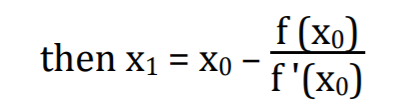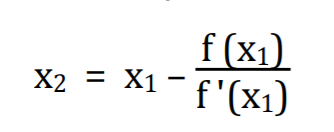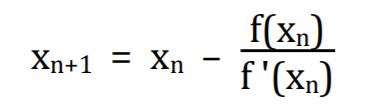Newton-Raphson's Method↪ The Newton-Raphson’s Method or Newton’s Method is a powerful technique for solving

equations numerically.

↪ Let f(x) be a well-behaved function and let x be a root of the equation f(x) = 0. We start

with an estimate x0
of x. From x0, we produce an improved estimate x1. From x1 we

produced a new estimate x
2. From x2, we produce a new estimate x3. We go on until we

are close enough to r or until it becomes
clear that we are getting nowhere. The above

general style of proceeding is called iterative. Of the many iterative root finding

procedures, the Newton Raphson’s Method, with its combination of simplicity and power,
is most widely used.

NEWTON RAPHSON’S ITERATION

↪ Let f(x) = 0 be an equation and x be its root. Let x0 be a good estimate of x then for a

point (x, y) sufficiently close to it the function can be approximated by its tangent line.↪ This tangent line crosses the x-axis when y = 0. Denote this new value of x by x1↪ ﻿This x intercept gives a better approximation to the function's root than the original

guess.

The next estimate x2 is obtained from x1 in exactly the same way as x1 was obtained from        x0↪ Continue in this way, if xn is the current estimate, then the next estimate xn + 1

is given by﻿